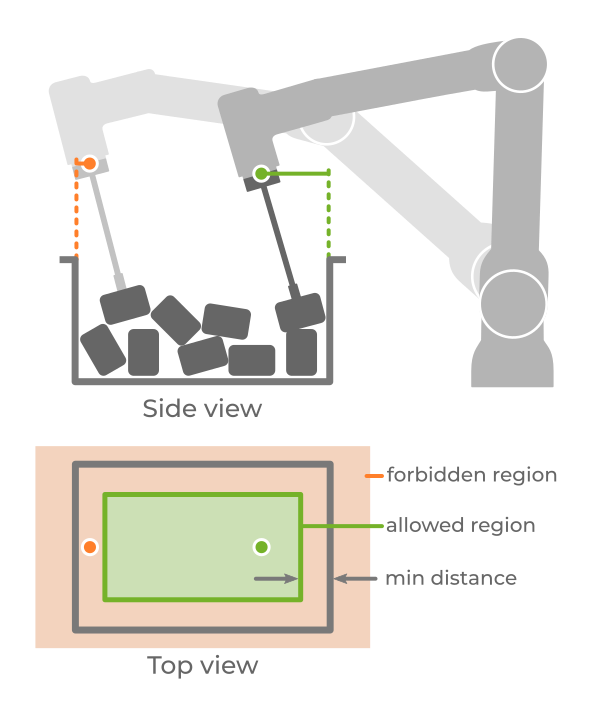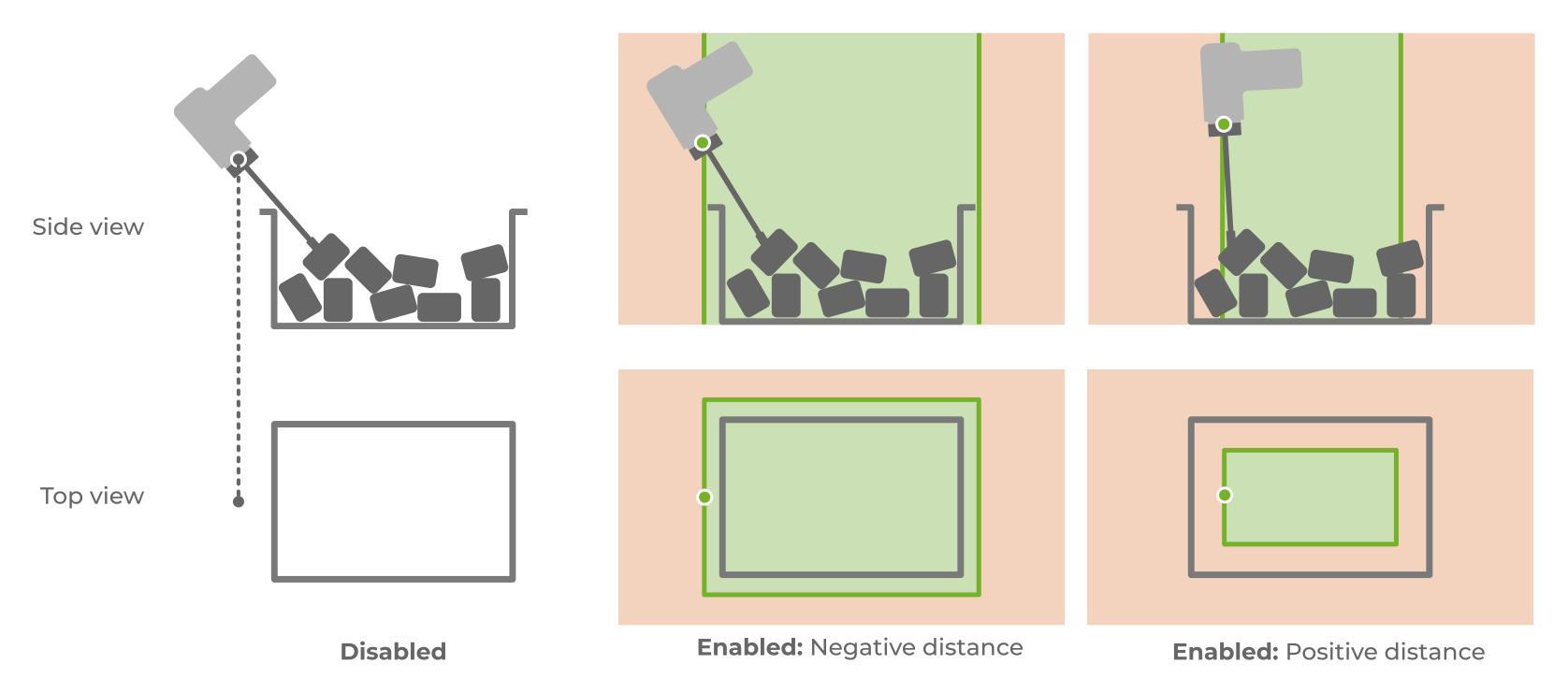# Minimum distance between robot flange and ROI

It is sometimes desired that the robot flange, when picking objects, does not deviate too much laterally above the ROI. The minimum distance between the robot flange and the ROI is such that objects that don’t respect it are labelled as unpickable. The below example visualizes the region of allowed flange positions, as well as two picks, one that doesn’t satisfy this constraint (left, in orange), and one that does (right, in green).This distance can be positive or negative. Negative values allow the flange to be in a region larger than the ROI, as viewed from the top. Positive values, on the other hand, allow it to be in a region smaller than the ROI. Note that only the lateral distance is checked, that is, the flange height is ignored in this constraint.

The example below shows a pick where this constraint is initially disabled (left), and how it changes upon enabling the constraint and setting a negative (center) and positive (right) distance.This contraint, along with the maximum robot flange tilt, limits the robot workspace used for picking on the Pickit side. If robot constraints (kinematics, joint limits, safety planes, etc.) are compatible with this allowed region, the resulting robot motions end up being reachable, predictable, and potentially faster.

Multiple pick points, symmetry axes and the tool model’s flexible pick orientation are explored to find a pick point that satisfies this constraint. If this is not possible, the pick point is labeled as unpickable. In the example below, we pick a part with a tool that has tilt flexibility, represented as a green circular section around the pick point.

• When possible, we prefer picks that don’t leverage tilt flexibility, so this is what Pickit first checks for (below left).

• This pick doesn’t satify the minimum distance between robot flange and ROI, and would in fact also be unreachable by the robot.

• Pickit then explores the tool’s tilt flexibility and finds a pick where the flange is at an acceptable distance from the ROI (below right). This pick is sent to the robot.